# Frank Solutions for Class 10 Maths Chapter 11 Matrices

Frank Solutions for Class 10 Maths Chapter 11 Matrices are available here. When students refer to the most comprehensive and detailed Frank Solutions for Class 10 Maths, they enhance their exam preparation. We, at BYJU’S, have prepared step-by-step solutions with detailed explanations. We advise students who wish to score good marks in Maths, to go through these solutions and strengthen their knowledge

Chapter 11 – Matrices defines a matrix as a set of numbers arranged in rows and columns so as to form a rectangular array. The numbers are called the elements, or entries, of the matrix. This chapter contains topics related to the classification of matrices and finding the unknown values in the matrices. So we suggest students practise Frank Solutions for Class 10 Maths to get more marks in Maths.

## Download the PDF of Frank Solutions for Class 10 Maths Chapter 11 Matrices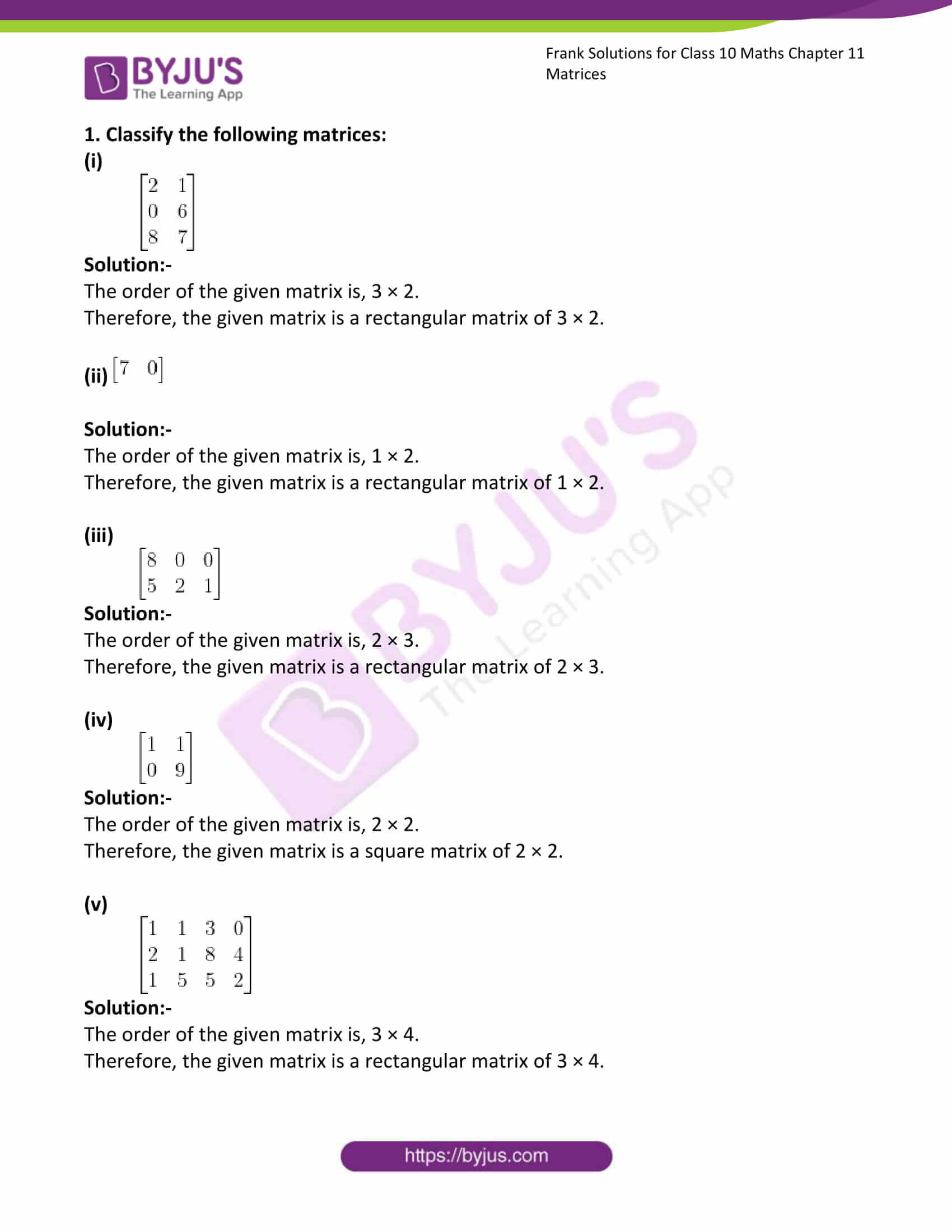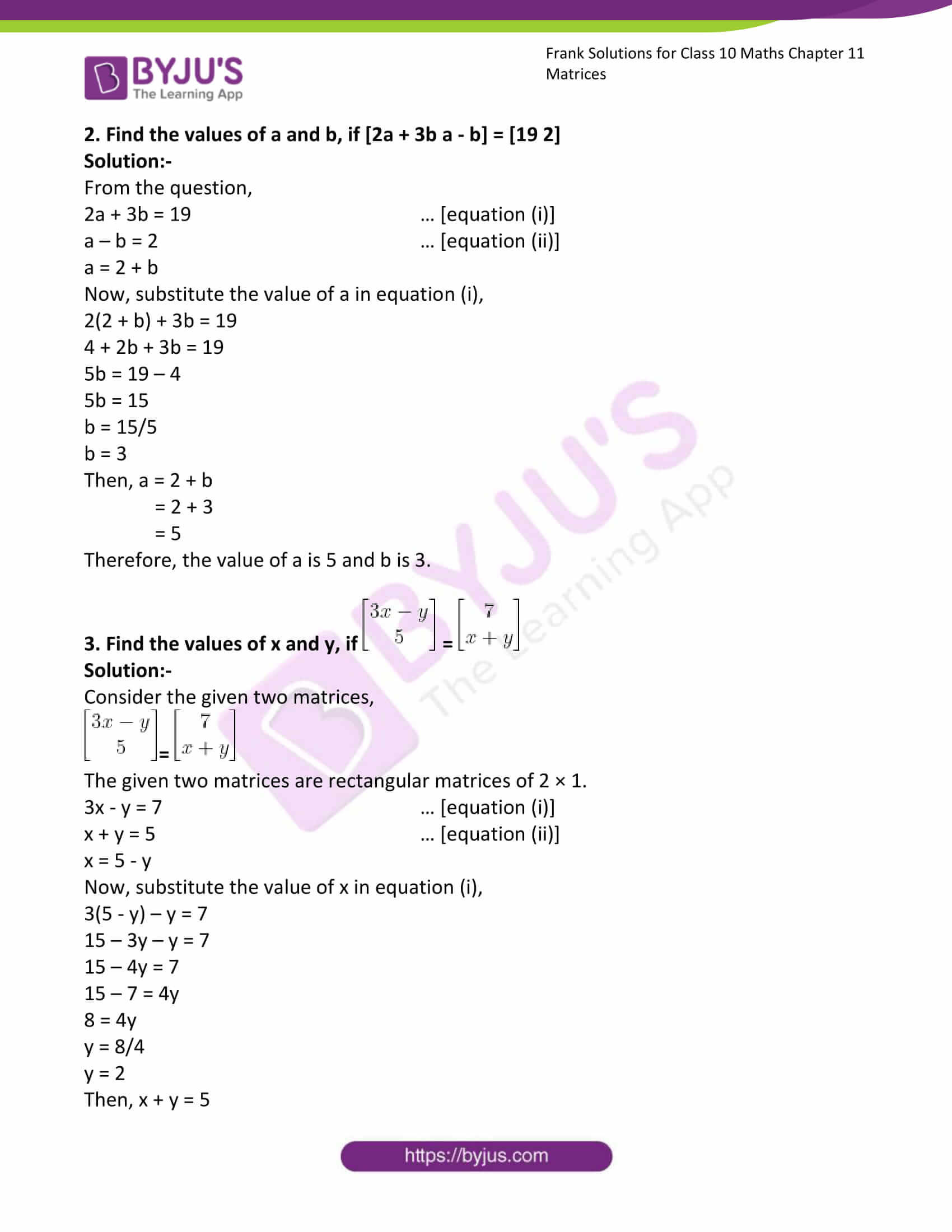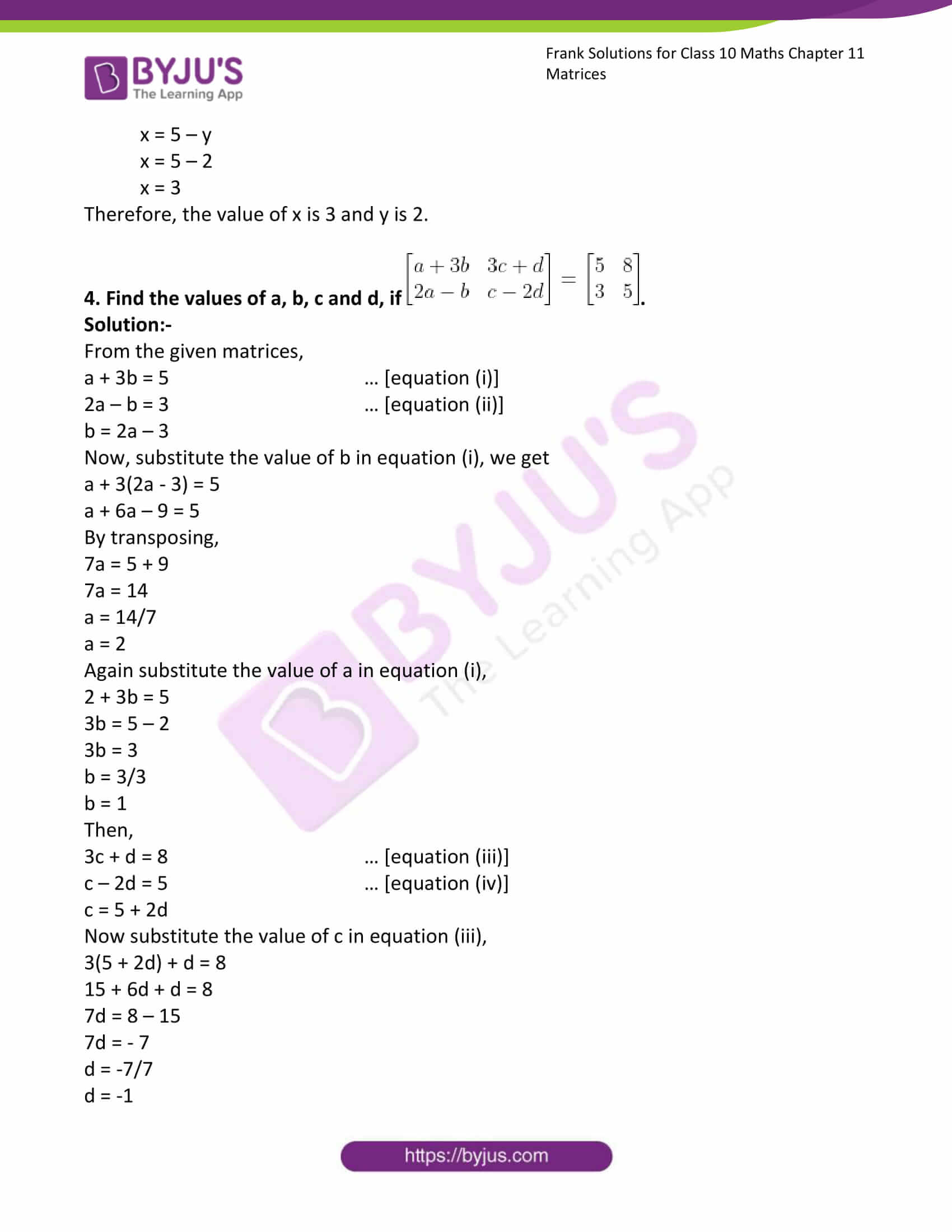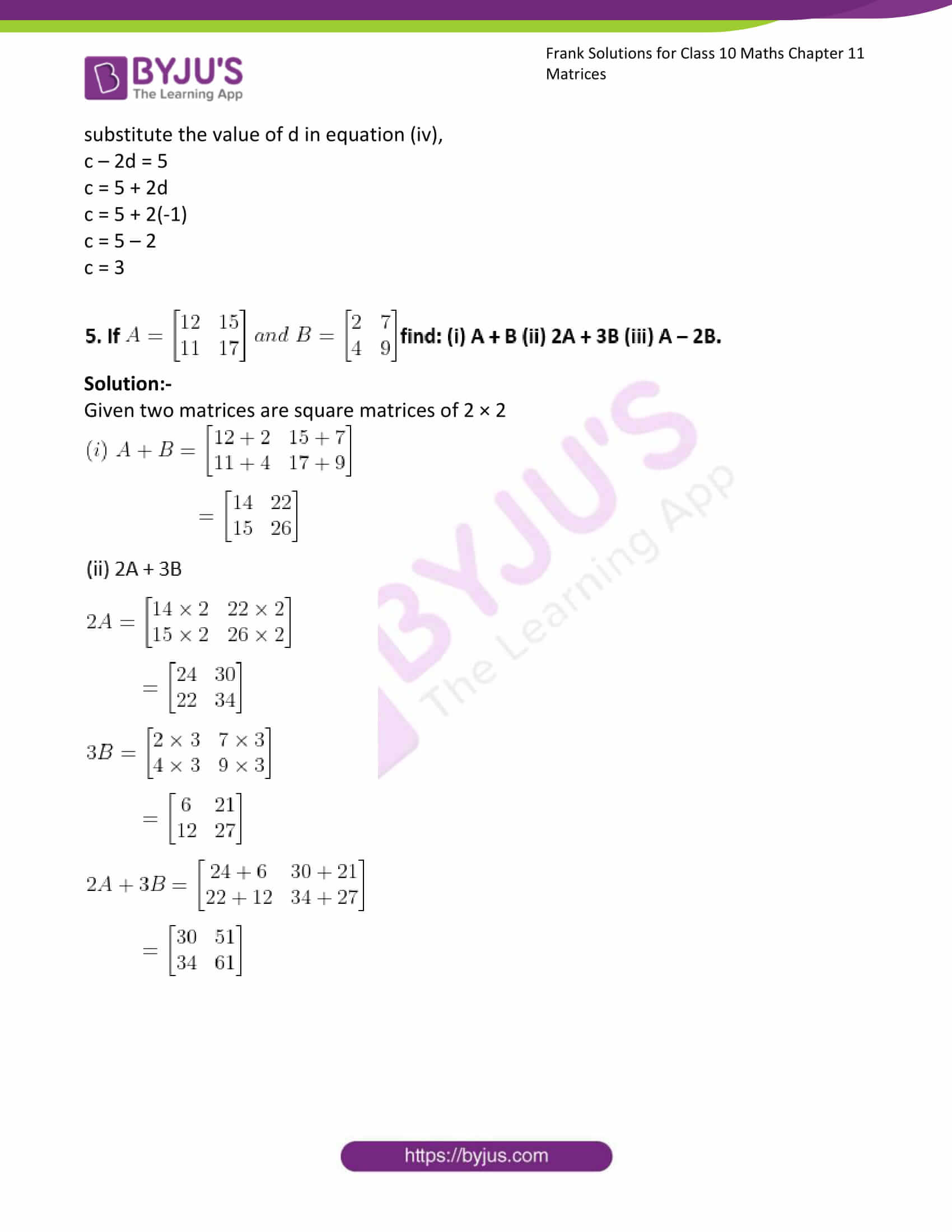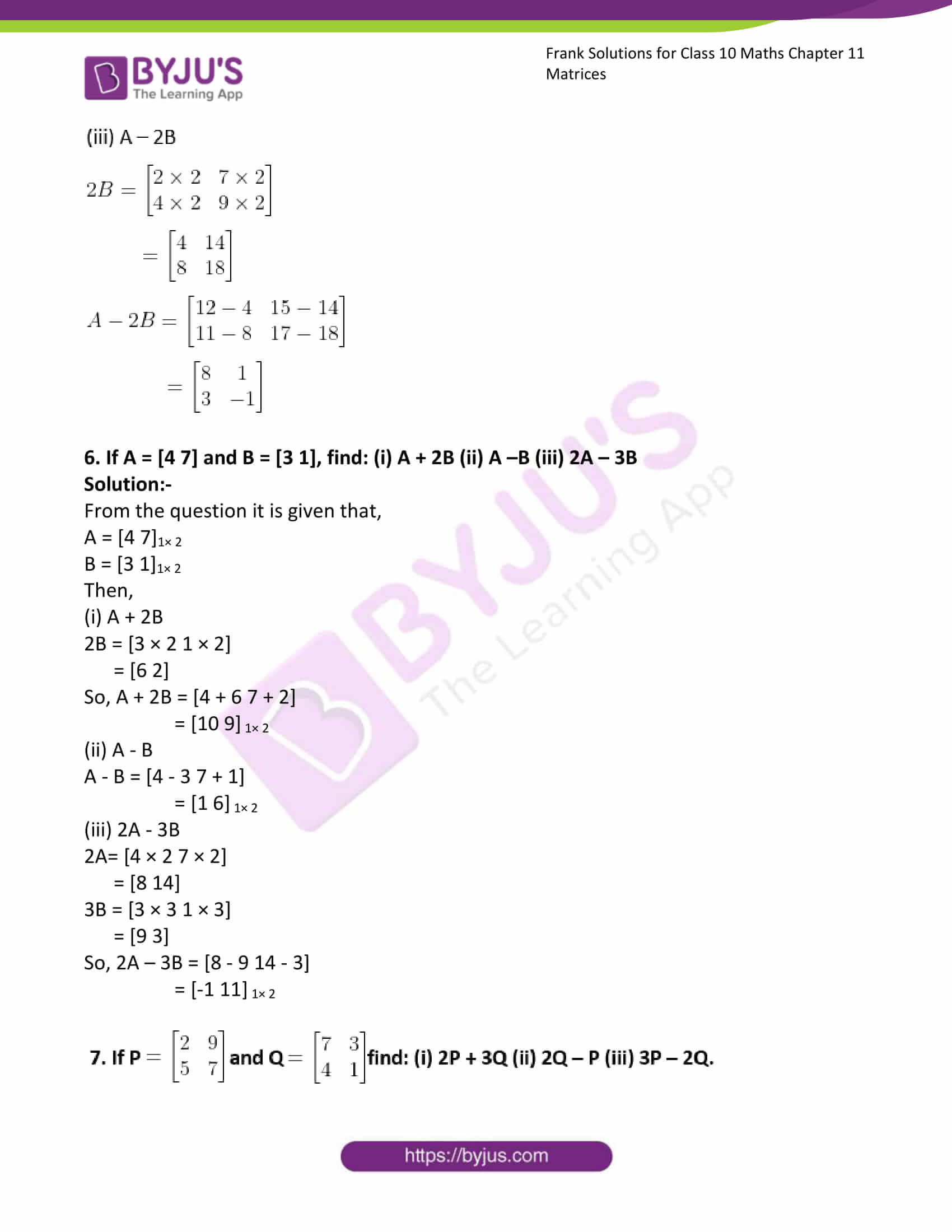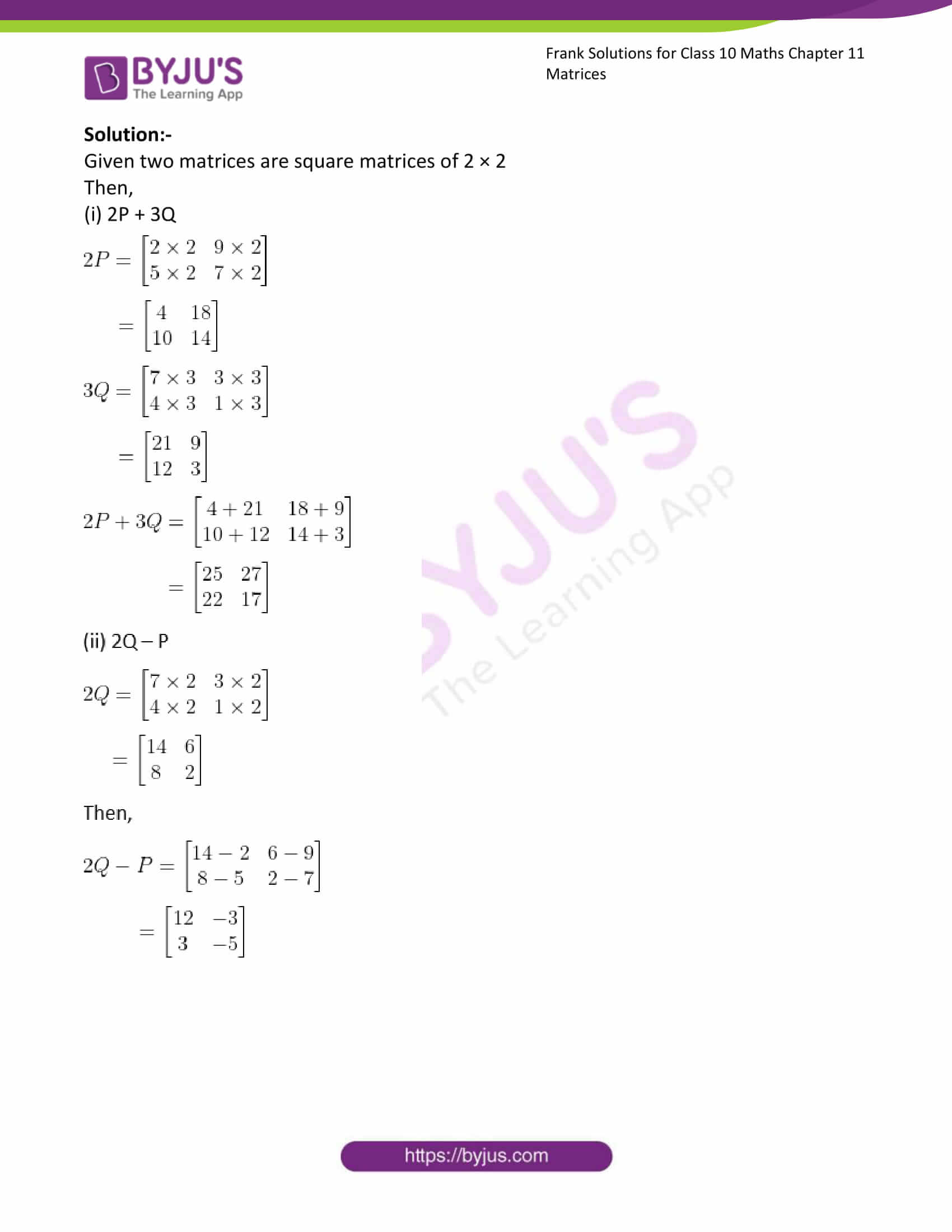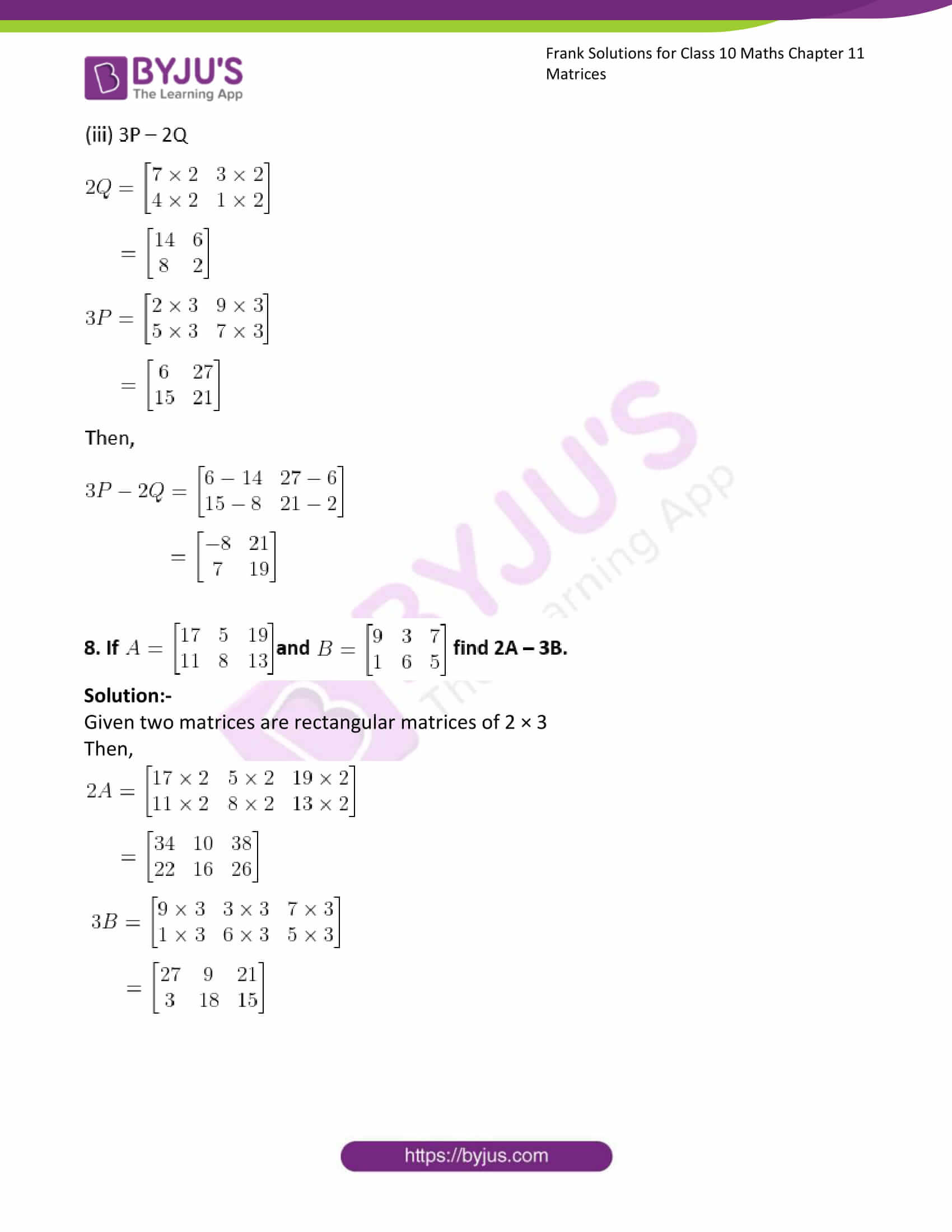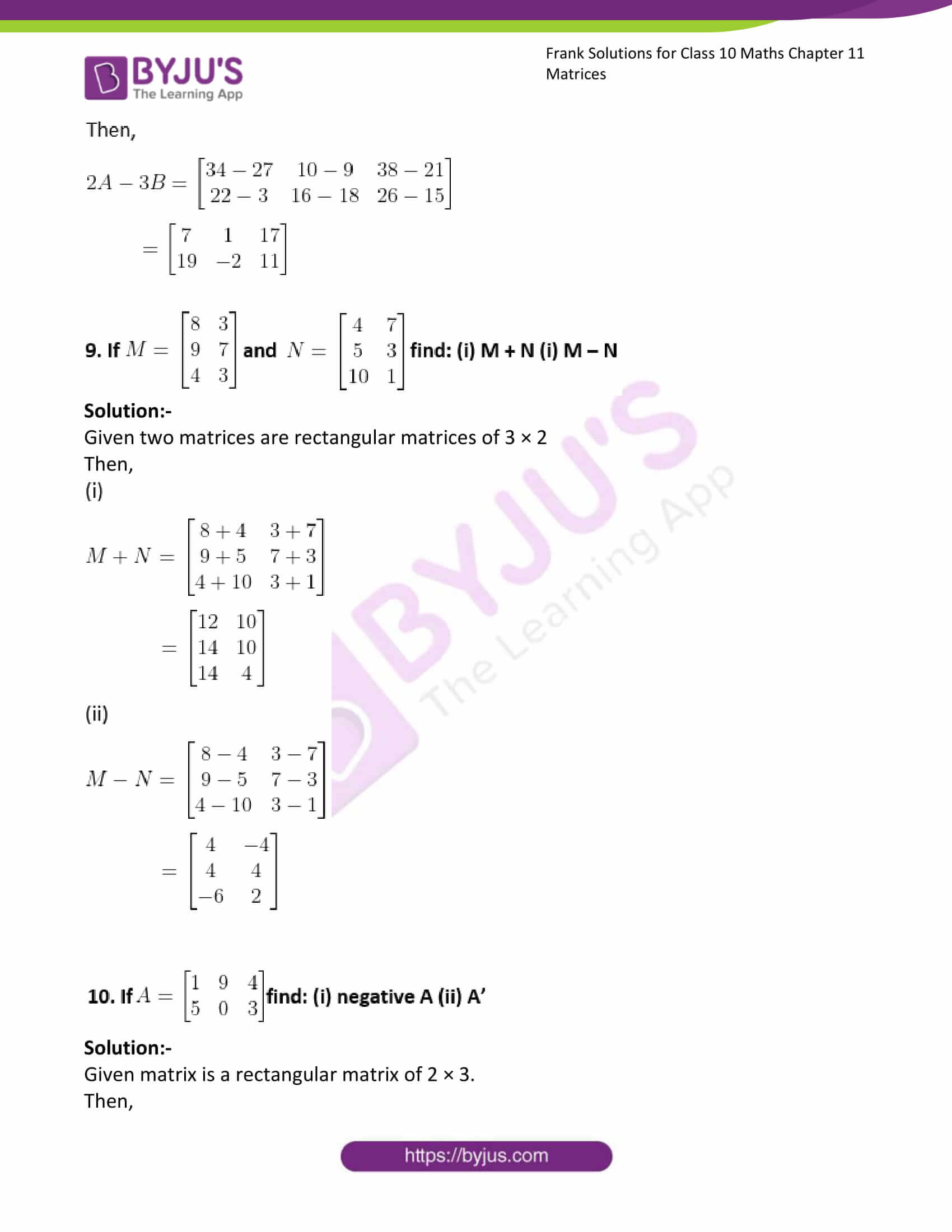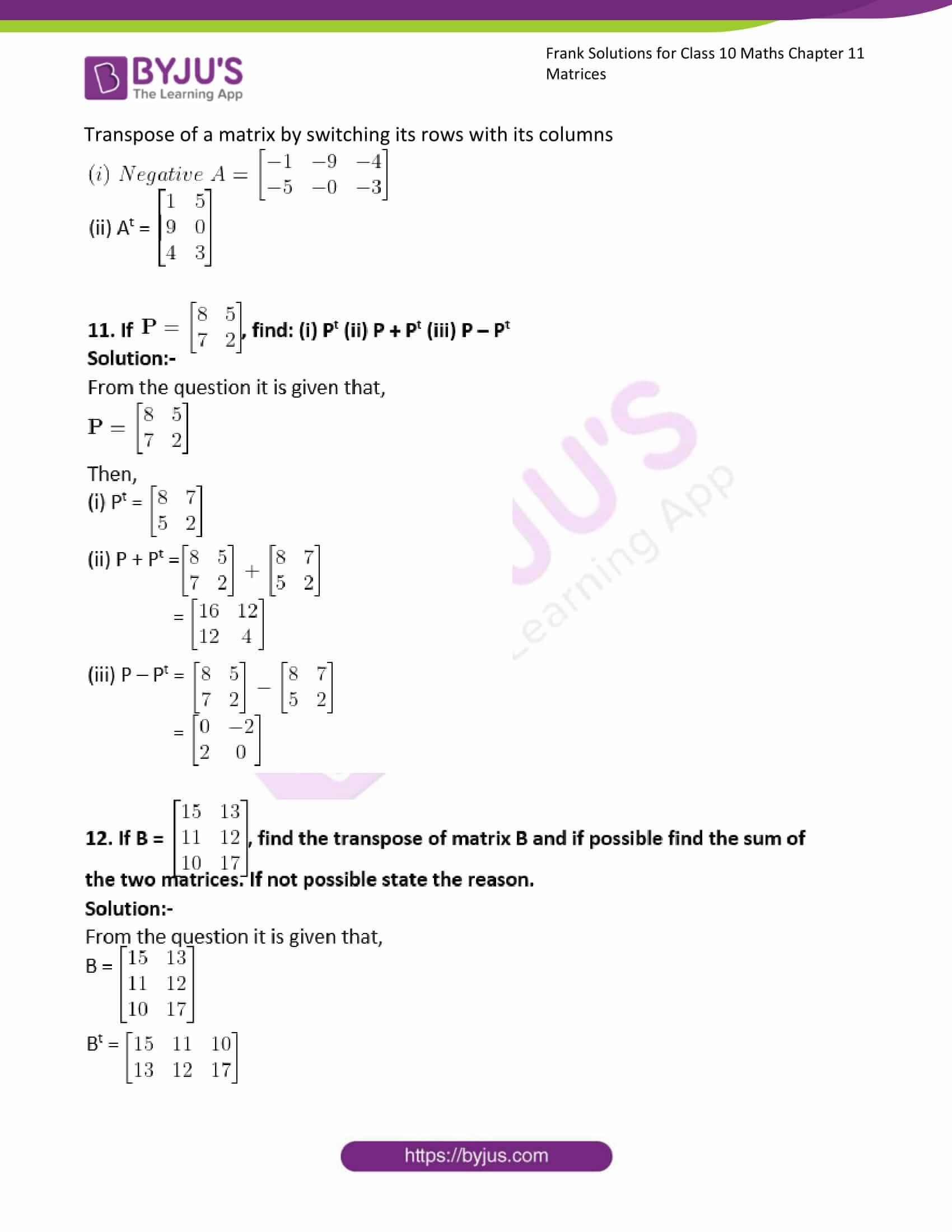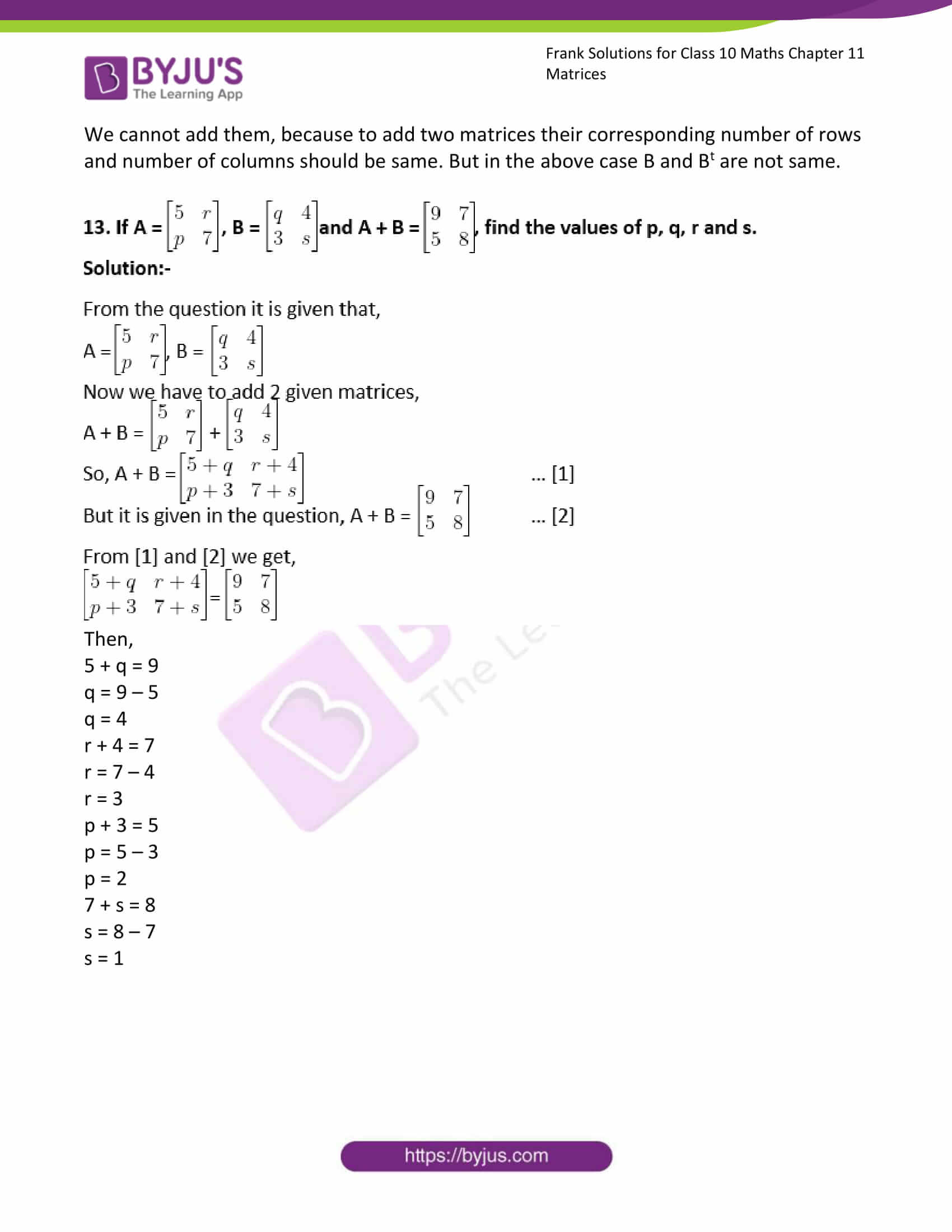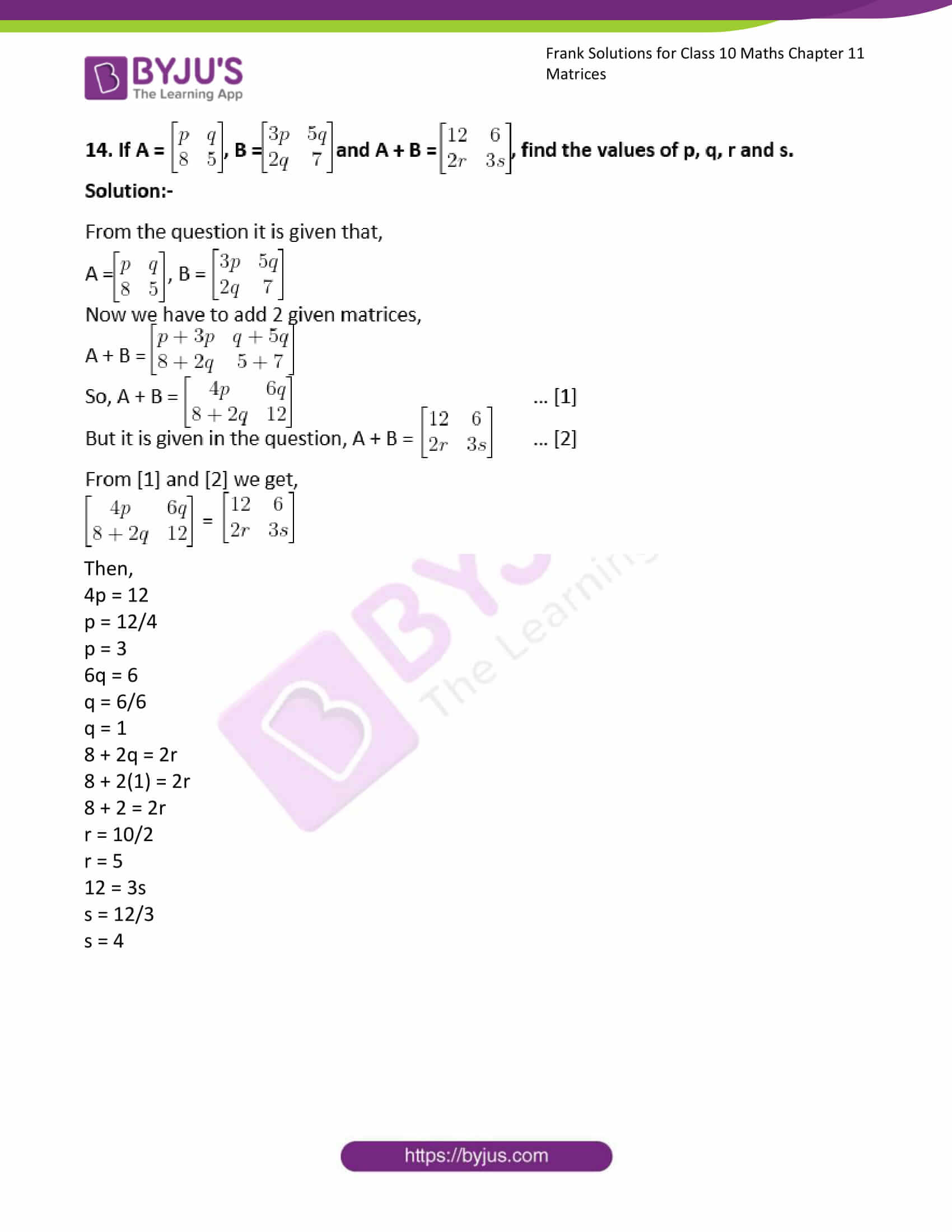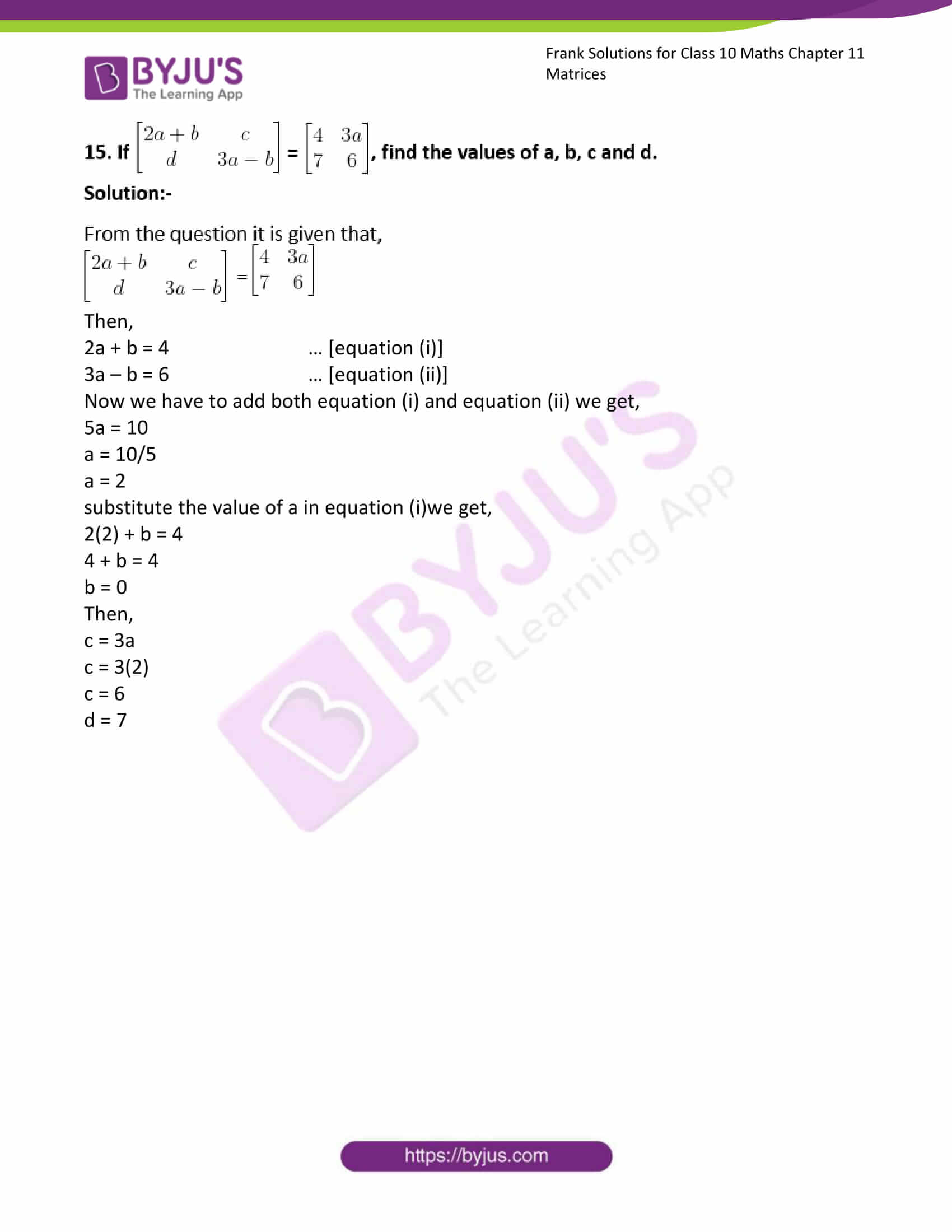### Access Answers to Frank Solutions for Class 10 Maths Chapter 11 Matrices

1. Classify the following matrices:

(i)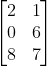Solution:-

The order of the given matrix is, 3 × 2.

Therefore, the given matrix is a rectangular matrix of 3 × 2.

(ii)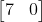Solution:-

The order of the given matrix is, 1 × 2.

Therefore, the given matrix is a rectangular matrix of 1 × 2.

(iii)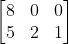Solution:-

The order of the given matrix is, 2 × 3.

Therefore, the given matrix is a rectangular matrix of 2 × 3.

(iv)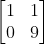Solution:-

The order of the given matrix is, 2 × 2.

Therefore, the given matrix is a square matrix of 2 × 2.

(v)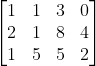Solution:-

The order of the given matrix is, 3 × 4.

Therefore, the given matrix is a rectangular matrix of 3 × 4.

2. Find the values of a and b, if [2a + 3b a – b] = [19 2]

Solution:-

From the question,

2a + 3b = 19 … [equation (i)]

a – b = 2 … [equation (ii)]

a = 2 + b

Now, substitute the value of a in equation (i),

2(2 + b) + 3b = 19

4 + 2b + 3b = 19

5b = 19 – 4

5b = 15

b = 15/5

b = 3

Then, a = 2 + b

= 2 + 3

= 5

Therefore, the value of a is 5 and b is 3.

3. Find the values of x and y, if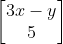=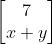Solution:-

Consider the given two matrices,=The given two matrices are rectangular matrices of 2 × 1.

3x – y = 7 … [equation (i)]

x + y = 5 … [equation (ii)]

x = 5 – y

Now, substitute the value of x in equation (i),

3(5 – y) – y = 7

15 – 3y – y = 7

15 – 4y = 7

15 – 7 = 4y

8 = 4y

y = 8/4

y = 2

Then, x + y = 5

x = 5 – y

x = 5 – 2

x = 3

Therefore, the value of x is 3 and y is 2.

4. Find the values of a, b, c and d, if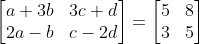.

Solution:-

From the given matrices,

a + 3b = 5 … [equation (i)]

2a – b = 3 … [equation (ii)]

b = 2a – 3

Now, substitute the value of b in equation (i), we get

a + 3(2a – 3) = 5

a + 6a – 9 = 5

By transposing,

7a = 5 + 9

7a = 14

a = 14/7

a = 2

Again substitute the value of a in equation (i),

2 + 3b = 5

3b = 5 – 2

3b = 3

b = 3/3

b = 1

Then,

3c + d = 8 … [equation (iii)]

c – 2d = 5 … [equation (iv)]

c = 5 + 2d

Now substitute the value of c in equation (iii),

3(5 + 2d) + d = 8

15 + 6d + d = 8

7d = 8 – 15

7d = – 7

d = -7/7

d = -1

substitute the value of d in equation (iv),

c – 2d = 5

c = 5 + 2d

c = 5 + 2(-1)

c = 5 – 2

c = 3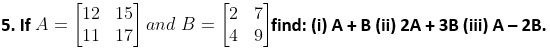Solution:-

Given two matrices are square matrices of 2 × 2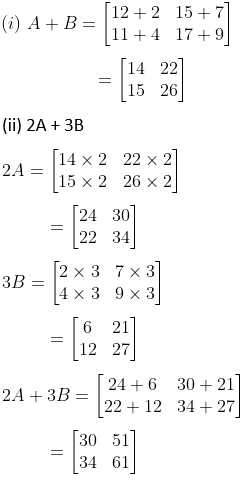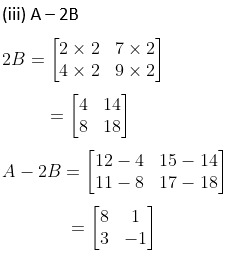6. If A = [4 7] and B = [3 1], find: (i) A + 2B (ii) A –B (iii) 2A – 3B

Solution:-

From the question it is given that,

A = [4 7]1× 2

B = [3 1]1× 2

Then,

(i) A + 2B

2B = [3 × 2 1 × 2]

= [6 2]

So, A + 2B = [4 + 6 7 + 2]

= [10 9] 1× 2

(ii) A – B

A – B = [4 – 3 7 + 1]

= [1 6] 1× 2

(iii) 2A – 3B

2A= [4 × 2 7 × 2]

= [8 14]

3B = [3 × 3 1 × 3]

= [9 3]

So, 2A – 3B = [8 – 9 14 – 3]

= [-1 11] 1× 2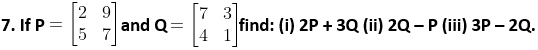Solution:-

Given two matrices are square matrices of 2 × 2

Then,

(i) 2P + 3Q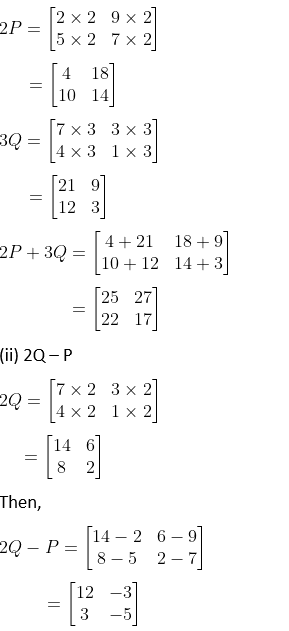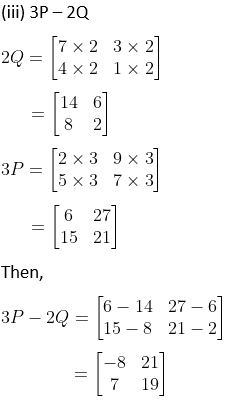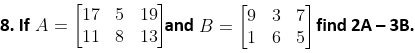Solution:-

Given two matrices are rectangular matrices of 2 × 3

Then,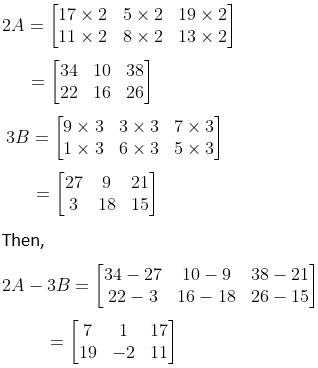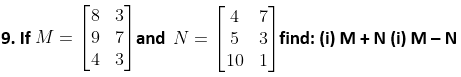Solution:-

Given two matrices are rectangular matrices of 3 × 2

Then,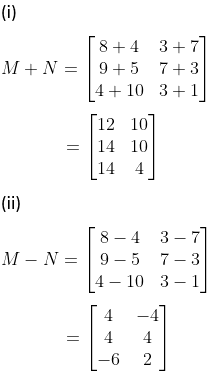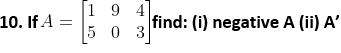Solution:-

Given matrix is a rectangular matrix of 2 × 3.

Then,

Transpose of a matrix by switching its rows with its columns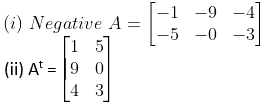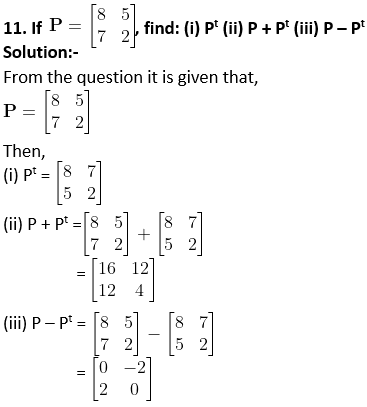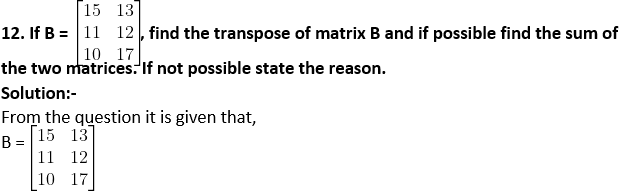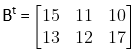We cannot add them, because to add two matrices their corresponding number of rows and number of columns should be same. But in the above case B and Bt are not same.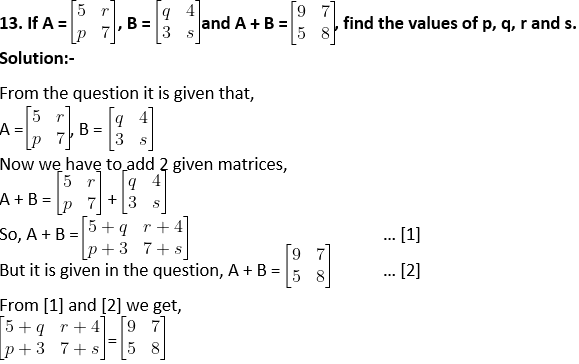Then,

5 + q = 9

q = 9 – 5

q = 4

r + 4 = 7

r = 7 – 4

r = 3

p + 3 = 5

p = 5 – 3

p = 2

7 + s = 8

s = 8 – 7

s = 1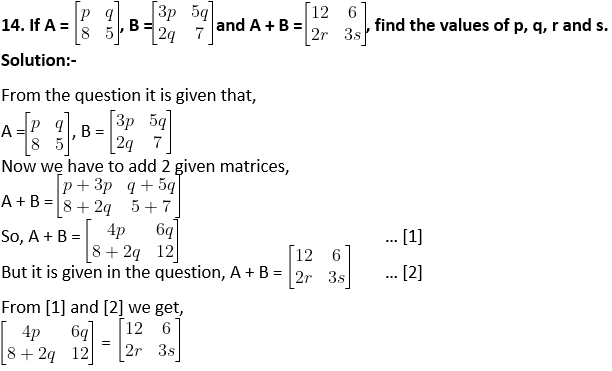Then,

4p = 12

p = 12/4

p = 3

6q = 6

q = 6/6

q = 1

8 + 2q = 2r

8 + 2(1) = 2r

8 + 2 = 2r

r = 10/2

r = 5

12 = 3s

s = 12/3

s = 4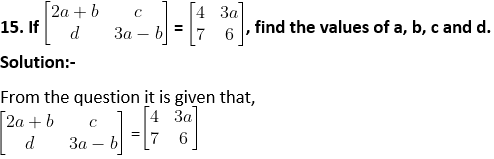Then,

2a + b = 4 … [equation (i)]

3a – b = 6 … [equation (ii)]

Now we have to add both equation (i) and equation (ii); we get,

5a = 10

a = 10/5

a = 2

Substitute the value of a in equation (i); we get,

2(2) + b = 4

4 + b = 4

b = 0

Then,

c = 3a

c = 3(2)

c = 6

d = 7